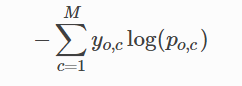# Pytorch formula for NLL Loss

Hi,

I was wondering why the negative log likelihood function (`NLLLoss()`) in torch.nn expected a target. The `torch.nn.NLLLoss()` uses `nll_loss(input, target, weight=self.weight, ignore_index=self.ignore_index, reduction=self.reduction)` in his forward call. If NLL has the format :, why is the target vector needed to compute this, and not just the output of our nn.Softmax() layer?

Thanks,

JP

In your formula I assume `y` gives the target class prediction. Have a look at the nn.CrossEntropyLoss docs to see the applied formula.

So the formula below describes the CrossEntropyLoss function implemented in Pytorch.

From what I understand, this function never uses the label (or target) of the sample to compute the probability output for `class`, since `x[class]` refers to the probability of our sample belonging to `class`, and sum(exp `(x[j])` is just the sum over the exponential of outputs for all classes. So where does Pytorch actually use the label of the sample in this loss function? I feel I’m missing something

1 Like

By indexing `x` with `class` you are indeed using your target. Without the target you won’t know which logit to use.

1 Like

Got it, thanks!

Related to this, I’m actually trying to tweak this loss function. I’d like to replace the target (`y_o,c`) in the loss function below by the prediction probability output for a specific class `p_o,c`, basically turning thisinto thisI tried simply replacing the `target` argument by `outputs` again when calling a `CrossEntropyLoss()` object, but Pytorch expects a datatype `torch.long`, not a `torch.float` as it is the case with `outputs`. Do you have any idea how I could obtain this new loss function?

Thanks,

JP

If you are looking for label smoothing, this thread might have an interesting code snippet.
Alternatively, you could just write out the formula.
I’m not sure, how `p_o,c` is defined, but I guess it should be the probability of class `c` for output `o`?

``````-1. * (target * F.log_softmax(x, 1)[target]).sum()
``````

If we’re talking mini-batch training, `p_o,c` would be an array of size (batch_size x num_classes), representing for each sample in th mini-batch it’s probability distribution over all possible classes. My concern was that I wanted a backpropagatable loss function. I’ll try that line and come back to you.

I think this is incorrect and nll_loss already assumes that you pass log(probabilities). You test this by giving it just probabilities (onehot format with values in range [0, 1]) and it will give you negative values.

2 Likes

I ended up modifying the function a bit for my needs, but it worked! Thanks